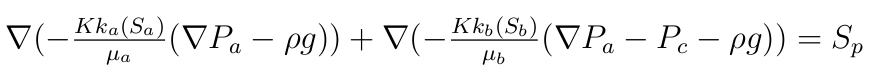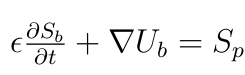# Resolution method

From the previous equations we obtained equation on the pressure :The pressure in the phase b is obtained by subtraction.

And we resolved the equation of the saturation of the phase b only :The saturation of the phase a is obtained by subtraction.

In the developed two-phase flow  solver we used a method called IMPES (Implicit Pressure Explicit Saturation). In this method the equation on the pressure is resolved in an implicit manner. Moreover the saturation of the liquid phase is resolved explicitly.

Then we resolve the equations of pressure and saturation separately. The positive point of this method is that we gain time of calculation, however the IMPES method could create in certain configurations instabilities during the saturation transport.